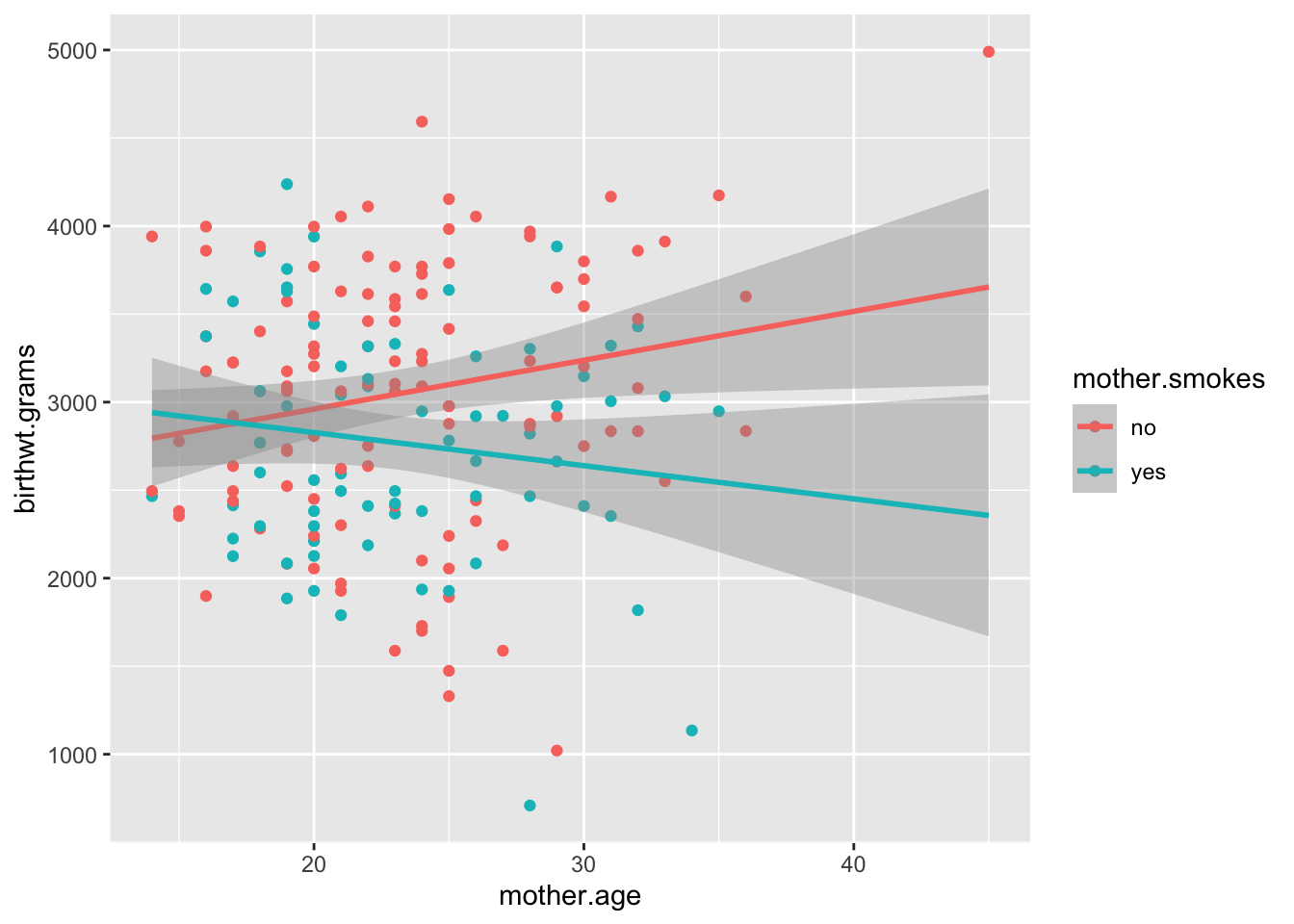### Learning objectives

In today’s Lab you will gain practice with the following concepts from today’s class:

• Interpreting linear regression coefficients of numeric covariates
• Interpreting linear regression coefficients of categorical variables
• Fitting linear regression models with interaction terms
• Interpreting linear regression coefficients of interaction terms

library(tidyverse)
## ── Attaching packages ─────────────────────────────────────────────────── tidyverse 1.2.1 ──
## ✔ ggplot2 3.3.2     ✔ purrr   0.3.3
## ✔ tibble  2.1.3     ✔ dplyr   0.8.3
## ✔ tidyr   1.0.0     ✔ stringr 1.4.0
## ✔ readr   1.3.1     ✔ forcats 0.4.0
## Warning: package 'ggplot2' was built under R version 3.6.2
## ── Conflicts ────────────────────────────────────────────────────── tidyverse_conflicts() ──
## ✖ dplyr::lag()    masks stats::lag()

### Interaction terms in regression

(a) Run a linear regression to better understand how birthweight varies with the mother’s age and smoking status (do not include interaction terms).

# Run regression model
birthwt.lm <- lm(birthwt.grams ~ mother.age + mother.smokes, data = birthwt)

# Output coefficients table
summary(birthwt.lm)
##
## Call:
## lm(formula = birthwt.grams ~ mother.age + mother.smokes, data = birthwt)
##
## Residuals:
##      Min       1Q   Median       3Q      Max
## -2119.98  -442.66    52.92   532.38  1690.74
##
## Coefficients:
##                  Estimate Std. Error t value Pr(>|t|)
## (Intercept)      2791.224    240.950  11.584   <2e-16 ***
## mother.age         11.290      9.881   1.143    0.255
## mother.smokesyes -278.356    106.987  -2.602    0.010 *
## ---
## Signif. codes:  0 '***' 0.001 '**' 0.01 '*' 0.05 '.' 0.1 ' ' 1
##
## Residual standard error: 717.2 on 186 degrees of freedom
## Multiple R-squared:  0.04299,    Adjusted R-squared:  0.0327
## F-statistic: 4.177 on 2 and 186 DF,  p-value: 0.0168

(b) What is the coefficient of mother.age in your regression? How do you interpret this coefficient?

coef(birthwt.lm)["mother.age"]
## mother.age
##   11.28961
age.coef <- round(coef(birthwt.lm)["mother.age"], 1)

Note: This solution uses inline code chunks. The coefficient is 11.3. This means that among mothers with the same smoking status, each additional year of age is on average associated with a 11.3g increase in birthweight. However, this coefficient is not statistically significant, so there may be no association between mother’s age and birth weight.

(c) How many coefficients are estimated for the mother’s smoking status variable? How do you interpret these coefficients?

coef(birthwt.lm)["mother.smokesyes"]
## mother.smokesyes
##        -278.3561
smoke.coef <- abs(round(coef(birthwt.lm)["mother.smokesyes"], 1))

Note: This solution uses inline code chunks. There is just one coefficient estimated. This coefficient gives us the average difference in birthweight between mothers that smoke and mother’s that don’t, in a model that adjusts for the effect of mother’s age. That is, after we adjust for the effect of age, smoking leads to an average 278.4 decrease in birthweight.

(d) What does the intercept mean in this model?

The intercept is the (extrapolated) estimated birth weight of a baby born to a non-smoking mother who is 0 years of age. Note that this value doesn’t really make sense because mothers can’t be 0 years of age when they give birth.

(e) Using ggplot, construct a scatterplot with birthweight on the y-axis and mother’s age on the x-axis. Color the points by mother’s smoking status, and add smoking status-specific linear regression lines using the stat_smooth layer.

library(ggplot2)

# Note fullrange = TRUE is used here to extend the 'mother.smokes = yes' line beyond the maximum age (35) in this group
qplot(data = birthwt, x = mother.age, y = birthwt.grams, colour = mother.smokes) + stat_smooth(method = "lm", fullrange = TRUE)
## geom_smooth() using formula 'y ~ x'(f) Do the regression lines plotted in part (e) correspond to the model you fit in part (a)? How can you tell?

The regression lines shown here do not correspond to the model in part (a). The lines in the plot have different slopes depending on whether the mother smokes or not. This is an interaction effect between mother’s smoking status and mother’s age. By contrast, the model in part (a) allows only for different intercepts, and assumes a common slope for both smoking categories.

(g) Fit a linear regression model that now models potential interactions between mother’s age and smoking status in their effect on birthweight.

# Note: this is the same as ~ mother.smokes + mother.age + mother.smokes*mother.age
birthwt.lm.interact <- lm(birthwt.grams ~ mother.smokes*mother.age, data = birthwt)

summary(birthwt.lm.interact)
##
## Call:
## lm(formula = birthwt.grams ~ mother.smokes * mother.age, data = birthwt)
##
## Residuals:
##      Min       1Q   Median       3Q      Max
## -2189.27  -458.46    51.46   527.26  1521.39
##
## Coefficients:
##                             Estimate Std. Error t value Pr(>|t|)
## (Intercept)                  2406.06     292.19   8.235 3.18e-14 ***
## mother.smokesyes              798.17     484.34   1.648   0.1011
## mother.age                     27.73      12.15   2.283   0.0236 *
## mother.smokesyes:mother.age   -46.57      20.45  -2.278   0.0239 *
## ---
## Signif. codes:  0 '***' 0.001 '**' 0.01 '*' 0.05 '.' 0.1 ' ' 1
##
## Residual standard error: 709.3 on 185 degrees of freedom
## Multiple R-squared:  0.06909,    Adjusted R-squared:  0.054
## F-statistic: 4.577 on 3 and 185 DF,  p-value: 0.004068

(h) Interpret your model. Is the interaction term statistically significant? What does it mean?

# p-value for interaction coefficient
pval.interact <- coef(summary(birthwt.lm.interact))["mother.smokesyes:mother.age", "Pr(>|t|)"]

slope.nosmoke <- coef(birthwt.lm.interact)["mother.age"]

slope.smoke <- coef(birthwt.lm.interact)["mother.age"] + coef(birthwt.lm.interact)["mother.smokesyes:mother.age"]

The estimated interaction term between mother’s age and smoking status (estimated for mother.smokes = yes) is negative, and statistically significant at the 0.05 level (p-value = 0.0238896). This means that the slope (with age) among mothers that smoke is much smaller than the slope among mother’s that don’t. Indeed, among mothers that don’t smoke, for every additional year of age the average birthweight increases by 27.7313782g on average. Among mothers that do smoke, for every additional year of age the average birthweight decreases by 18.8405365g on average